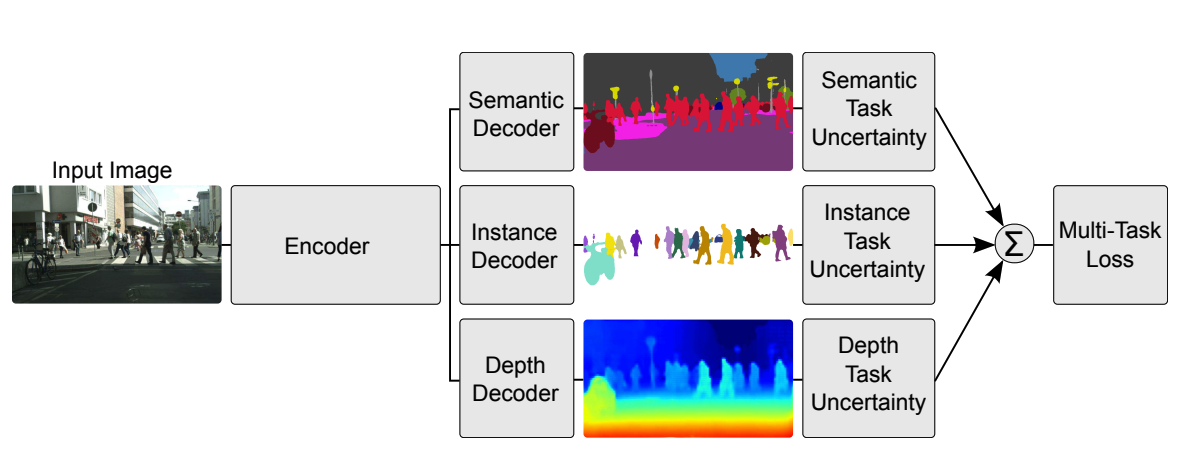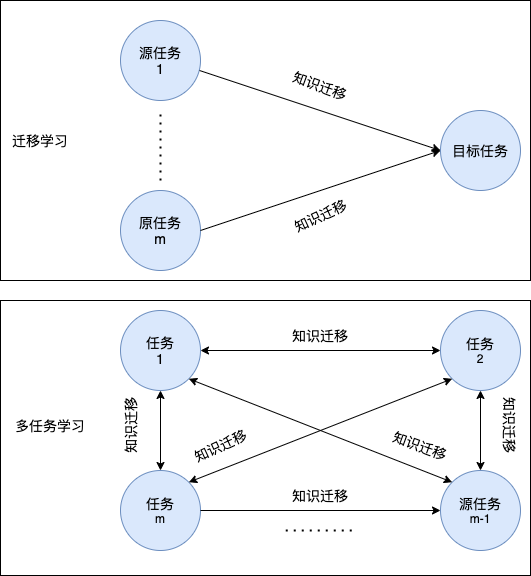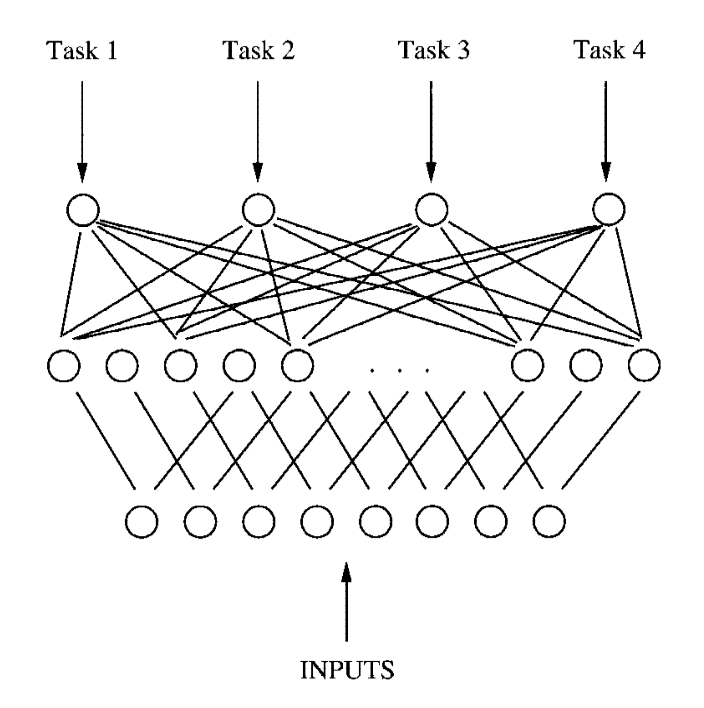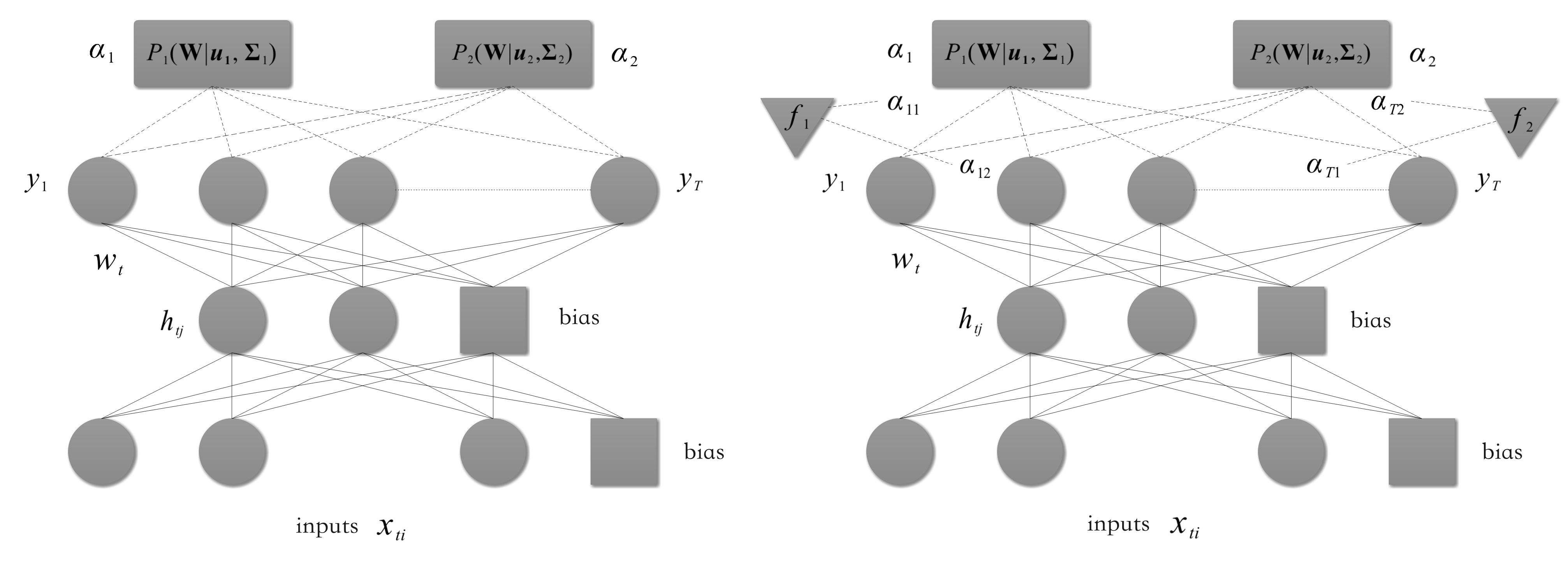# 基于正则化的多任务学习

## 1.导言## 2、多任务学习简介

### 2.1 多任务学习：迁移学习和知识表示的延伸

• 迁移学习可能有多个源域；而多任务学习没有源域而只有多个目标域。
• 迁移学习注重提升目标任务性能，并不关心源任务的性能（知识由源任务$$\rightarrow$$目标任务；而多任务学习旨在提高所有任务的性能(知识在所有任务间相互传递)。## 3、基于正则化的多任务学习

### 3.1 基于正则化的多任务学习的形式表述

\begin{aligned} \underset{\textbf{W}}{\min} & \sum_{t=1}^{T}\mathbb{E}_{(\bm{x_{ti}, y_{ti})\sim \mathcal{D}_t}}[L(y_{ti}, f(\bm{x}_{ti}; \bm{w}_t))]\\ & = \sum_{t=1}^{T} [\frac{1}{m_t}\sum_{i=1}^{m_t}L(y_{ti}, f(\bm{x}_{ti}; \bm{w}_t))] \end{aligned} \tag{1}

（此处$$\textbf{W}=(\bm{w}_1,\bm{w}_2,...,\bm{w}_T)$$为所有任务参数构成的矩阵）

\begin{aligned} \underset{\textbf{W}}{\min} & \sum_{t=1}^{T} [\frac{1}{m_t}\sum_{i=1}^{m_t}L(y_{ti}, f(\bm{x}_{ti}; \bm{w}_t))]+\lambda g(\textbf{W})\\ & = f(\textbf{W}) + \lambda g(\textbf{W}) \end{aligned} \tag{2}

### 3.2.1 基于特征的多任务学习

a. 特征变换\begin{aligned} & \underset{\textbf{A},\mathbf{U},\bm{b}}{\min} \sum_{t=1}^{T} [\frac{1}{m_t}\sum_{i=1}^{m_t}L(y_{ti}, \langle \bm{a}_t, \mathbf{U}^T\bm{x}_{ti} \rangle+b_t)]+\lambda ||\textbf{A}||_{2,1}^2 \\ & \text{s. t. } \quad \mathbf{U}\mathbf{U}^T = \mathbf{I} \end{aligned} \tag{3}

\begin{aligned} & \underset{\textbf{A},\mathbf{U},\bm{b}}{\min} \sum_{t=1}^{T} [\frac{1}{m_t}\sum_{i=1}^{m_t}L(y_{ti}, \langle \bm{a}_t, \mathbf{U}^T\bm{x}_{ti} \rangle+b_t)] \\ & \text{s. t. } \quad ||\bm{a}_i||_{1} \leqslant \lambda , \quad i=1,2,..,m\\ &\quad\quad\quad||\bm{u}_j||_{2}\leqslant 1, \quad j=1,2,.., d^{'}，表示第j列 \end{aligned} \tag{4}

b. 联合特征学习（joint feature learning）

$\underset{\textbf{W}, \bm{b}}{\min} \sum_{t=1}^{T} [\frac{1}{m_t}\sum_{i=1}^{m_t}L(y_{ti}, \langle \bm{w}_t, \bm{x}_{ti} \rangle + b_{t})]+\lambda ||\textbf{W}||_{p, q} \tag{5}$

$$l_{p, q}$$正则化的特例是$$l_{2, 1}$$(Obozinski等人，2006、2010)和$$l_{\infin}$$无穷正则化（Liu等人，2009b）。$$l_{2, 1}$$正则化中采用$$l{2, 1}$$范数$$||\textbf{W}||_{2, 1} = \sum_{i=1}^{d}||\bm{w}^{i}||_2$$（此处$$d$$为特征维度，$$\bm{w}^i$$$$\textbf{W}$$$$i$$行），$$l_{\infin,1}$$正则化采用$$l_{\infin, 1}$$范数$$||\mathbf{W}||_{\infin, 1}=\sum_{i=1}^d||\bm{w}^i||_{\infin}= \sum_{i=1}^d\underset{1\leqslant t \leqslant T}{\text{max}}|w_{jt}|$$，即先对每一行方向求绝对值最大，然后再沿着行方向求和（注意区分这个和矩阵的$$\infin$$范数，求和与求最大的顺序是不一样的!这里相当于求向量的无穷范数之和)）。为了获得对所有特征都有用的更紧凑的子集，Gong等人(2013)提出了上限$$l_{p, 1}$$惩罚项$$\sum_{i=1}^{d}\min(||\bm{w}^i||_p, \theta)$$，当$$\theta$$足够大时它将退化为$$l_{p, 1}$$正则化。

### 3.2.2 基于模型的多任务监督学习

a. 共享子空间学习(shared subspace learning)

\begin{aligned} & \underset{\textbf{P},\mathbf{Q},\mathbf{\Theta},\bm{b}}{\min} \sum_{t=1}^{T} [\frac{1}{m_t}\sum_{i=1}^{m_t}L(y_{ti}, \langle \bm{p}_t + \mathbf{\Theta}^T \bm{q}_t, \bm{x}_{ti} \rangle + b_{t}]+\lambda ||\textbf{P}||_{F}^2 \\ & \text{s. t. } \quad \mathbf{\Theta}\mathbf{\Theta}^T = \mathbf{I} \end{aligned} \tag{6}

Chen等人(2009)通过为$$\mathbf{W}$$增加平方Frobenius正则化推广了这一模型（Frobenius范数表达式为$$||\mathbf{A}||_{F}= (\text{tr}(\mathbf{A}^T\mathbf{A}))^{1/2}=\left(\sum_{i=1}^m\sum_{j=1}^nA_{ij}^2 \right)^{1/2} = \left(\sum_{i=1}^{\min(d, T)}\sigma_i(\mathbf{A})^2\right)^{1/2}$$），并采用松弛技术将问题转换为了凸优化问题。

$\underset{\textbf{W}, \bm{b}}{\min} \sum_{t=1}^{T} [\frac{1}{m_t}\sum_{i=1}^{m_t}L(y_{ti}, \langle \bm{w}_t, \bm{x}_{ti} \rangle + b_{t}]+\lambda ||\textbf{W}||_{*} \tag{7}$

b. 聚类方法

Thrun等人（1996）提出了第一个任务聚类算法，它包含两个阶段。在第一阶段，该方法根据在单任务下单独学习得到的模型来聚类任务，确定不同的任务簇。在第二阶段，聚合同一个任务簇中的所有训练数据，以学习这些任务的模型。这种方法把任务聚类和模型参数学习分为了两个阶段，可能得不到最优解，因此后续工作都采用了同时学习任务聚类和模型参数的方法。
Bakker等人(2003)提出了一个多任务贝叶斯神经网络(multi-task Bayesian neural network)，其结构与我们前面所展现的多层神经网络相同，其亮点在于基于连接隐藏层和输出层的权重采用高斯混合模型（Gaussian mixture model）对任务进行分组。若给定数据集$$\mathcal{D} = \{D_t\}, t=1,...,T$$，设隐藏层维度为$$h$$，输出层维度为$$T$$$$\mathbf{W}\in \mathbb{R}^{T\times (h + 1)}$$代表隐藏层到输出层的权重矩阵(结合了偏置)。我们假定每个任务对应的权重向量$$\mathbf{w}_t$$($$\mathbf{W}$$的第$$t$$列)关于给定超参数独立同分布，我们假定第$$t$$个任务先验分布如下：

$\bm{w}_t \sim \mathcal{N}(\bm{w}_t | \bm{u}, \mathbf{\Sigma}) \tag{8}$

$\bm{w}_t \sim \sum_{c=1}^C \alpha_c \mathcal{N} (\bm{w}_t | \bm{u}_c, \mathbf{\Sigma}_c) \tag{9}$Xue等人（2007）根据模型参数应用Dirichlet过程（一种广泛用于数据聚类的贝叶斯模型）对任务进行分组。

\begin{aligned} & \underset{\textbf{W}, \bm{b}, \bm{\Sigma}}{\min} \sum_{t=1}^{T} [\frac{1}{m_t}\sum_{i=1}^{m_t}L(y_{ti}, \langle \bm{w}_t, \bm{x}_{ti} \rangle + b_{t})] +\lambda_1 \text{tr}(\textbf{W}\textbf{U}\textbf{W}^T) + \text{tr}(\textbf{W}\mathbf{\Pi}\mathbf{\Sigma}^{-1}\mathbf{\Pi}\mathbf{W}^T) \\ & \text{s.t.}\quad \alpha \mathbf{I} \preccurlyeq \mathbf{\Sigma} \preccurlyeq \beta \mathbf{I}, \text{tr}(\mathbf{\Sigma}) = \gamma \end{aligned} \tag{10}

Kang等人(2011)将式$$(3)$$所示的MTFL方法扩展到多个任务簇的情况下，其中每个任务簇中任务的学习模型是MTFL方法，其目标函数为：

\begin{aligned} & \underset{\textbf{W}, \bm{b}, \{\bm{Q}_c\}}{\min} \sum_{t=1}^{T} [\frac{1}{m_t}\sum_{i=1}^{m_t}L(y_{ti}, \langle \bm{w}_t, \bm{x}_{ti} \rangle + b_{t})] +\lambda \sum_{c=1}^{C}||\mathbf{W}\mathbf{Q}_c||_*^{2} \\ & \text{s.t.}\quad q_{ct} \in \{0, 1\} 且\sum_{c=1}^{C}\mathbf{Q}_c = \mathbf{I}, \space c=1,2,..,C \end{aligned} \tag{11}

$\mathbf{W} = \sum_{h=1}^H\mathbf{W}_h \tag{12}$

$$\mathbf{W}_h$$用于学习第$$h$$层次的任务簇。$$\mathbf{W}_h = (\bm{w}_{h,1},...,\bm{w}_{h,T})\in \mathbb{R}^{d\times T}$$，其中$$\bm{w}_{h, t}$$是在$$h$$层次中任务$$t$$的参数。该论文将最终的正则化的目标函数(论文称之为MeTaG方法)写为

\begin{aligned} & \underset{\textbf{W}}{\min} \sum_{t=1}^{T} [\frac{1}{m_t}\sum_{i=1}^{m_t}L(y_{ti}, \langle \sum_{h=1}^{H} \bm{w}_{h,t}, \bm{x}_{ti} \rangle + b_t)] + \sum_{h=1}^{H} \lambda_h \sum_{i<j}^{T}||\bm{w}_{h,i} - \bm{w}_{h,j}||_2 \end{aligned} \tag{13}

Kumar和Daume（2012）以及Barizilai和Crammer（2015）都提出了$$\mathbf{W} = \mathbf{L}\mathbf{S}(\mathbf{W} \in \mathbb{R}^{d\times T}, \mathbf{L} \in \mathbb{R}^{d\times C}, \mathbf{S} \in \mathbb{R}^{C\times T})$$的分解形式，其中$$\textbf{L}$$的列$$\bm{l}_c$$对应任务簇$$c$$的隐（latent）参数，$$\textbf{S}$$的列$$\bm{s}_t$$对应一组线性组合系数($$\bm{w}_t = \mathbf{L}\mathbf{s}_t$$，相当于$$s_t$$$$\mathbf{L}$$中各列进行线性组合，以得到$$\bm{w}_t$$)。这两种方法的目标函数可以统一为

\begin{aligned} & \underset{\textbf{W}}{\min} \sum_{t=1}^{T} [\frac{1}{m_t}\sum_{i=1}^{m_t}L(y_{ti}, \langle \textbf{L}\bm{s}_t, \bm{x}_{ti} \rangle + b_t)] + \lambda_1 h(\mathbf{S}) + \lambda_2||\mathbf{L}||_F^2 \end{aligned} \tag{14}

h(\mathbf{S}) = \left\{ \begin{aligned} & 0 \quad \mathbf{S} \in \{0, 1\}^{C\times T}, ||\bm{s}_t||_2=1 \\ & +\infin \quad 其他 \end{aligned} \right. \tag{15}

## 参考文献

•  Long M, Cao Z, Wang J, et al. Learning multiple tasks with multilinear relationship networks[J]. arXiv preprint arXiv:1506.02117, 2015.

•  Misra I, Shrivastava A, Gupta A, et al. Cross-stitch networks for multi-task learning[C]//Proceedings of the IEEE conference on computer vision and pattern recognition. 2016: 3994-4003.

•  Hashimoto K, Xiong C, Tsuruoka Y, et al. A joint many-task model: Growing a neural network for multiple nlp tasks[J]. arXiv preprint arXiv:1611.01587, 2016.

•  Kendall A, Gal Y, Cipolla R. Multi-task learning using uncertainty to weigh losses for scene geometry and semantics[C]//Proceedings of the IEEE conference on computer vision and pattern recognition. 2018: 7482-7491.

•  Evgeniou T, Pontil M. Regularized multi--task learning[C]//Proceedings of the tenth ACM SIGKDD international conference on Knowledge discovery and data mining. 2004: 109-117.

•  Zhou J, Chen J, Ye J. Malsar: Multi-task learning via structural regularization[J]. Arizona State University, 2011, 21.

•  Zhou J, Chen J, Ye J. Clustered multi-task learning via alternating structure optimization[J]. Advances in neural information processing systems, 2011, 2011: 702.

•  Ji S, Ye J. An accelerated gradient method for trace norm minimization[C]//Proceedings of the 26th annual international conference on machine learning. 2009: 457-464.

•  Caruana R. Multitask learning[J]. Machine learning, 1997, 28(1): 41-75

•  Evgeniou A, Pontil M. Multi-task feature learning[J]. Advances in neural information processing systems, 2007, 19: 41.

•  Argyriou A, Evgeniou T, Pontil M. Convex multi-task feature learning[J]. Machine learning, 2008, 73(3): 243-272.

•  Maurer A, Pontil M, Romera-Paredes B. Sparse coding for multitask and transfer learning[C]//International conference on machine learning. PMLR, 2013: 343-351.

•  Obozinski G, Taskar B, Jordan M. Multi-task feature selection[J]. Statistics Department, UC Berkeley, Tech. Rep, 2006, 2(2.2): 2.

•  Obozinski G, Taskar B, Jordan M I. Joint covariate selection and joint subspace selection for multiple classification problems[J]. Statistics and Computing, 2010, 20(2): 231-252.

•  Liu H, Palatucci M, Zhang J. Blockwise coordinate descent procedures for the multi-task lasso, with applications to neural semantic basis discovery[C]//Proceedings of the 26th Annual International Conference on Machine Learning. 2009: 649-656.

•  Gong P, Ye J, Zhang C. Multi-stage multi-task feature learning[J]. arXiv preprint arXiv:1210.5806, 2012.

•  Ando R K, Zhang T, Bartlett P. A framework for learning predictive structures from multiple tasks and unlabeled data[J]. Journal of Machine Learning Research, 2005, 6(11).

•  Chen J, Tang L, Liu J, et al. A convex formulation for learning shared structures from multiple tasks[C]//Proceedings of the 26th Annual International Conference on Machine Learning. 2009: 137-144.

•  Fazel M, Hindi H, Boyd S P. A rank minimization heuristic with application to minimum order system approximation[C]//Proceedings of the 2001 American Control Conference.(Cat. No. 01CH37148). IEEE, 2001, 6: 4734-4739.

•  Thrun S, O'Sullivan J. Discovering structure in multiple learning tasks: The TC algorithm[C]//ICML. 1996, 96: 489-497.

•  Bakker B J, Heskes T M. Task clustering and gating for bayesian multitask learning[J]. 2003.

•  Xue Y, Liao X, Carin L, et al. Multi-task learning for classification with dirichlet process priors[J]. Journal of Machine Learning Research, 2007, 8(1).

•  Zhou J, Chen J, Ye J. Clustered multi-task learning via alternating structure optimization[J]. Advances in neural information processing systems, 2011, 2011: 702.

•  Kang Z, Grauman K, Sha F. Learning with whom to share in multi-task feature learning[C]//ICML. 2011.

•  Han L, Zhang Y. Learning multi-level task groups in multi-task learning[C]//Twenty-Ninth AAAI Conference on Artificial Intelligence. 2015.

•  Kumar A, Daume III H. Learning task grouping and overlap in multi-task learning[J]. arXiv preprint arXiv:1206.6417, 2012.

•  Barzilai A, Crammer K. Convex multi-task learning by clustering[C]//Artificial Intelligence and Statistics. PMLR, 2015: 65-73.

•  杨强等. 迁移学习[M].机械工业出版社, 2020.

posted @ 2021-10-29 16:22  orion-orion  阅读(987)  评论(0编辑  收藏  举报